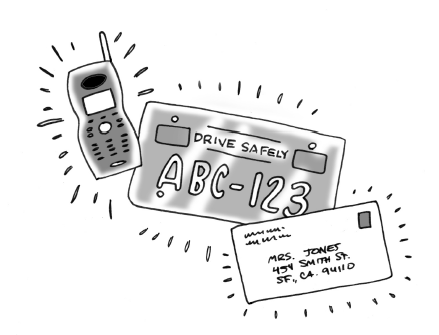### Home > CCG > Chapter Ch10 > Lesson 10.3.2 > Problem10-132

10-132.

In the past, many states had license plates composed of three letters followed by three digits ($0\text{ to }9$). Recently, many states have responded to the increased number of cars by adding one digit ($1\text{ to }9$) ahead of the three letters. How many more license plates of the second type are possible? What is the probability of being randomly assigned a license plate containing $\text{ALG }2$?Since repetition is allowed, the total number of license plates of the first type is
$26\cdot26\cdot26\cdot10\cdot10\cdot10 = 17,576,000$.

The total number of license plates of the second type is
$9\cdot26\cdot26\cdot26\cdot10\cdot10\cdot10 = 158,184,000$.

Find the difference.

Find the number of license plates containing $\text{ALG }2$.

$9\cdot1\cdot1\cdot1\cdot1\cdot10\cdot10 =$

$P(\text{ALG }2)=\frac{900}{158,184,000}=?$